# 机器学习笔记

## 一、机器学习的相关概念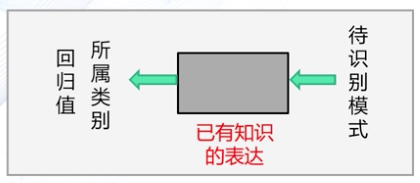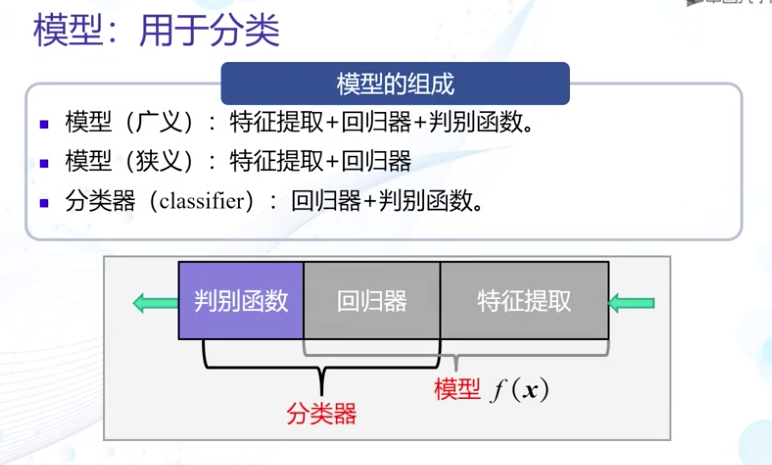## 二、特征的相关概念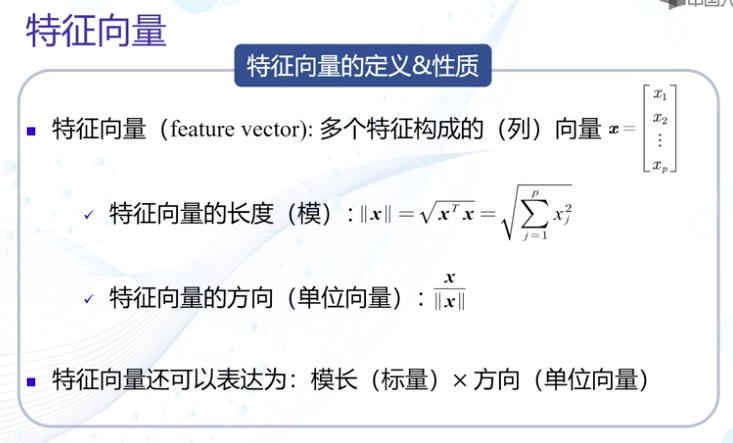• 点积
• $$x \cdot y = x^{T}y = y^{T}x = \sum^{p}_{j=1}{x_{j}y_{j}}$$
• 点积的结果是一个标量表达
• 点积是一个线性变换
• 几何意义：$$x \cdot y = |x||y|cos \theta$$
• 点积为0则表示两个向量共线，称为正交
• 向量夹角
• $$cos\theta = \frac{x \cdot y}{|x||y|}$$
• 投影
• $$x_{y} = |x|cos \theta$$
• $$x_{y}$$称为x到y上的投影，是x在y方向上的长度（标量
• 投影向量
• $$x_{y} = |x|cos\theta\frac{y}{|y|}$$
• $$x_{y}$$称为x在y上的分解，是x在y方向上的向量

• 两个特征向量之间的夹角表示在方向上的差异性
• x到y上的投影表示x分解到y上的程度，能分解的越多说明两者越相似
• 残差向量
• $$r_{x} = x-x_{y} = x-|x|cos\theta\frac{y}{|y|}$$
• 残差向量表示x分解到y上的投影向量与原向量x的误差
• 欧氏距离
• $$d(x,y) = (x-y)^{T}(x-y) = \sum^{p}_{j=1}{(x_{j}-y_{j})^{2}}$$
• 表示两个特征向量的相似程度

## 三、机器学习的评估方法与性能指标

• 测试误差
• 二类分类：真阳性(TP)，假阳性(FP)，真阴性(TN)，假阴性(FN)
• 多类分类：每类各自为正，非自身为负
• 准确度：判断值与真值完全一致的占比
• $$Accuracy=\frac{TP+TN}{Sum}$$
• 精度：阳性样本的准确度
• $$Precision = \frac{TP}{TP+FP}$$
• 召回率（真阳率）：所有阳性样本中被预测为阳性的比例
• $$Recall = TPR = \frac{TP}{TP+FN}$$
• 特异性
• $$Specificity = \frac{TN}{TN+FP}$$
• 假阳率
• $$FPR = 1-Specificity = \frac{FP}{TN+FP}$$
• F-Score：结合精度与召回率的指标
• $$F = \frac{(a^{2}+1) \times Precision \times Recall}{a^{2} \times Precision \times Recall}$$
• $$a=1$$，有$$F_{1} = \frac{2 \times Precision \times Recall}{Precision \times Recall}$$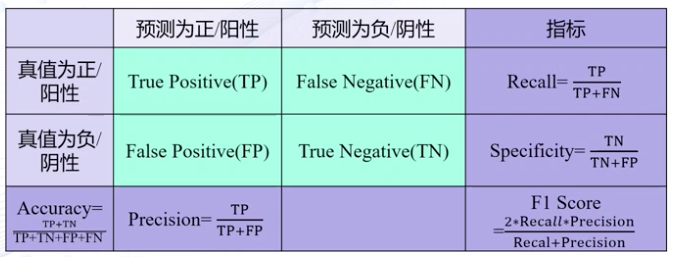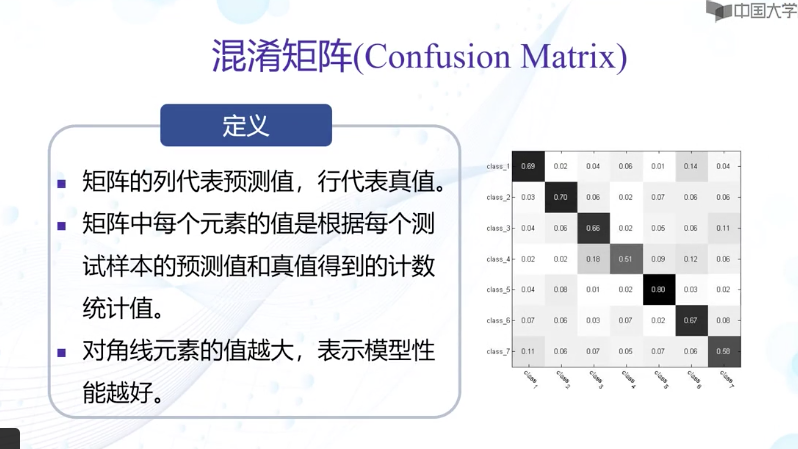• PR曲线
• 横轴：召回率（Recall）数值
• 纵轴：精度（Precision）数值
• 理想性能：右上角（1,1）处
• 召回率与精度越大性能越好，即越往右上角凸越好
•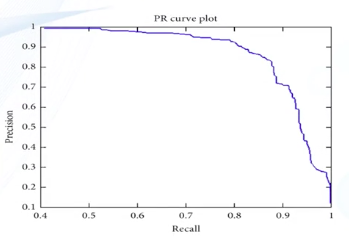• ROC曲线
• 横轴：假阳率（FPR）数值
• 纵轴：真阳率（TPR）数值
• 理想性能：左上角（0,1）处
• FPR越小TPR越大性能越好，即越往左上角凸越好
• 对角线：随机预测模型的ROC曲线
•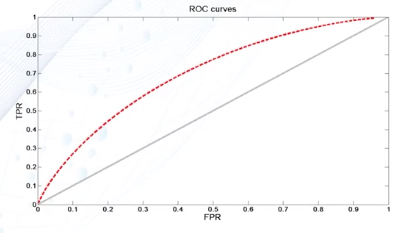• AUC曲线下方面积
• 面积越大性能越好
• $$AUC = 1$$表示这是完美的模型
• $$AUC > 0.5$$表示这是较好的模型
• $$AUC = 0.5$$表示该模型与随机预测模型是等效的
• $$AUC < 0.5$$表示比随机预测模型还差
posted @ 2020-05-06 04:12  Ace_Monster  阅读(332)  评论(0编辑  收藏  举报